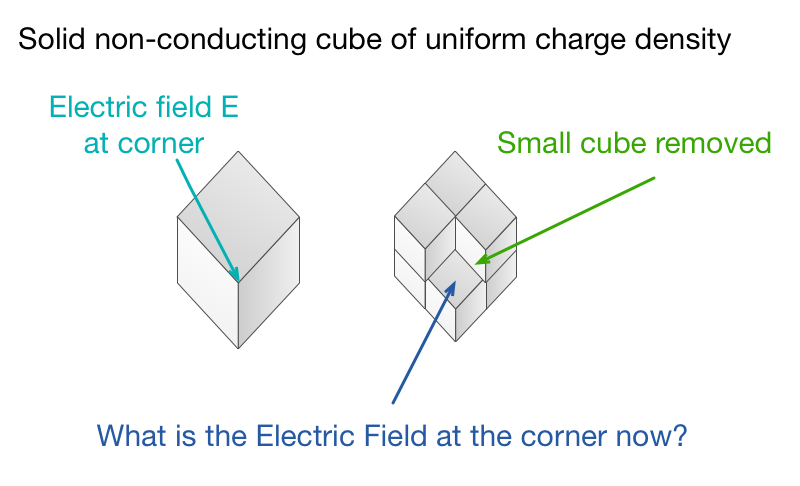# May be this is easier than you thinkA cube of side $a$ and uniform charge density $\rho$ has electric field $E$ at one of its corners.

A cube of side $\dfrac a2$ is removed from that corner. The electric field at this point is now $E_2$. Find $\dfrac{E_2}E$.

×

Problem Loading...

Note Loading...

Set Loading...